woebin_plot create plots of count distribution and positive probability for each bin. The binning informations are generates by woebin.

woebin_plot(bins, x = NULL, title = NULL, show_iv = TRUE,
line_value = "posprob", ...)

## Arguments

bins

A list of data frames. Binning information generated by woebin.

x

Name of x variables. Defaults to NULL. If x is NULL, then all columns except y are counted as x variables.

title

String added to the plot title. Defaults to NULL.

show_iv

Logical. Defaults to TRUE, which means show information value in the plot title.

line_value

The value displayed as line. Accepted values are 'posprob' and 'woe'. Defaults to positive probability.

...

## Value

A list of binning graphics.

woebin, woebin_ply, woebin_adj

## Examples

# Load German credit data
data(germancredit)

# Example I
bins1 = woebin(germancredit, y="creditability", x="credit.amount")
#> ℹ Creating woe binning ...
#> ✔ Binning on 1000 rows and 2 columns in 00:00:00

p1 = woebin_plot(bins1)
print(p1)
#> $credit.amount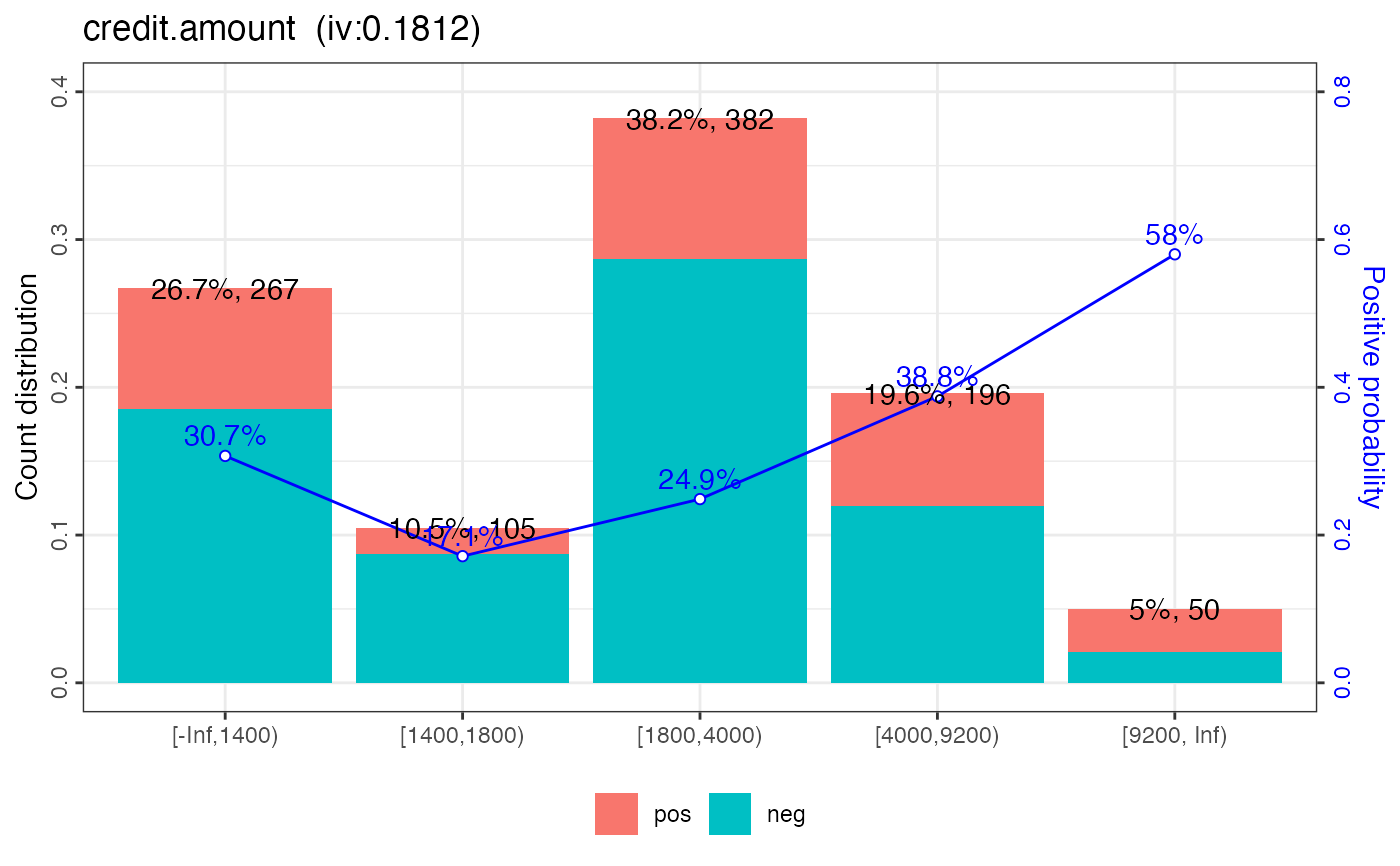#> # modify line value p1_w = woebin_plot(bins1, line_value = 'woe') print(p1_w) #>$credit.amount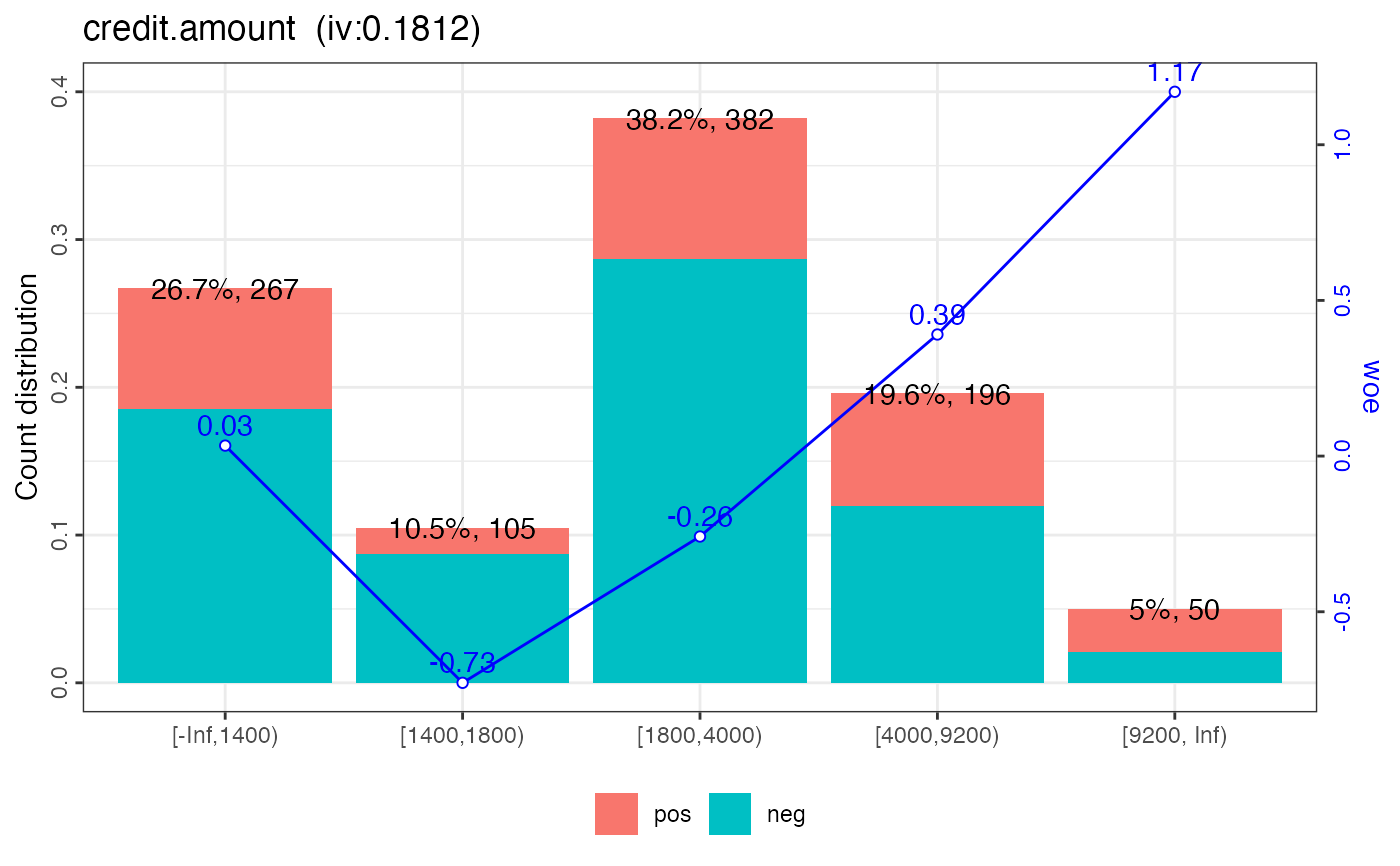#>

# modify colors
p1_c = woebin_plot(bins1, line_color='#FC8D59', bar_color=c('#FFFFBF', '#99D594'))
print(p1_c)
#> $credit.amount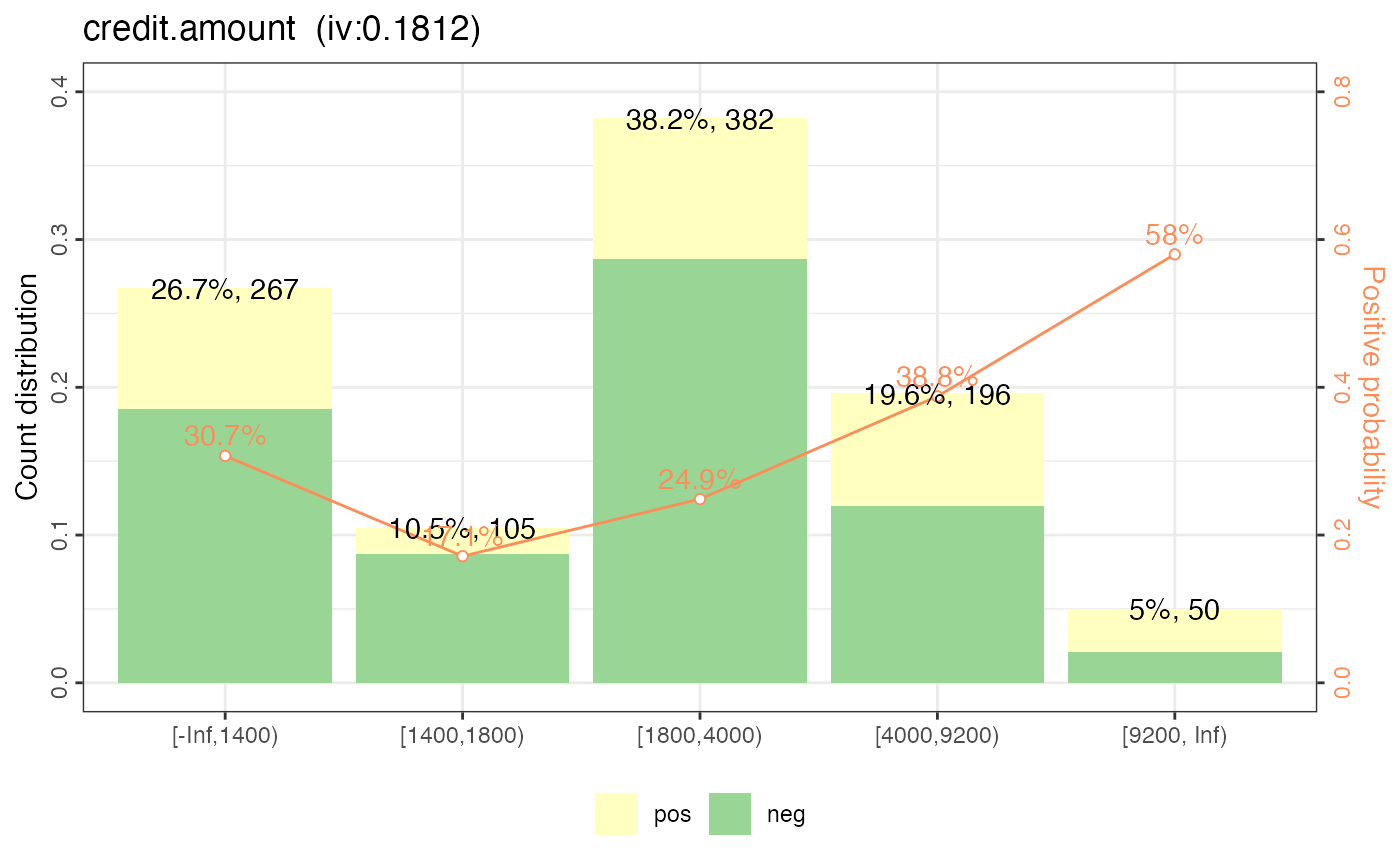#> # show iv, line value, bar value p1_iv = woebin_plot(bins1, show_iv = FALSE) print(p1_iv) #>$credit.amount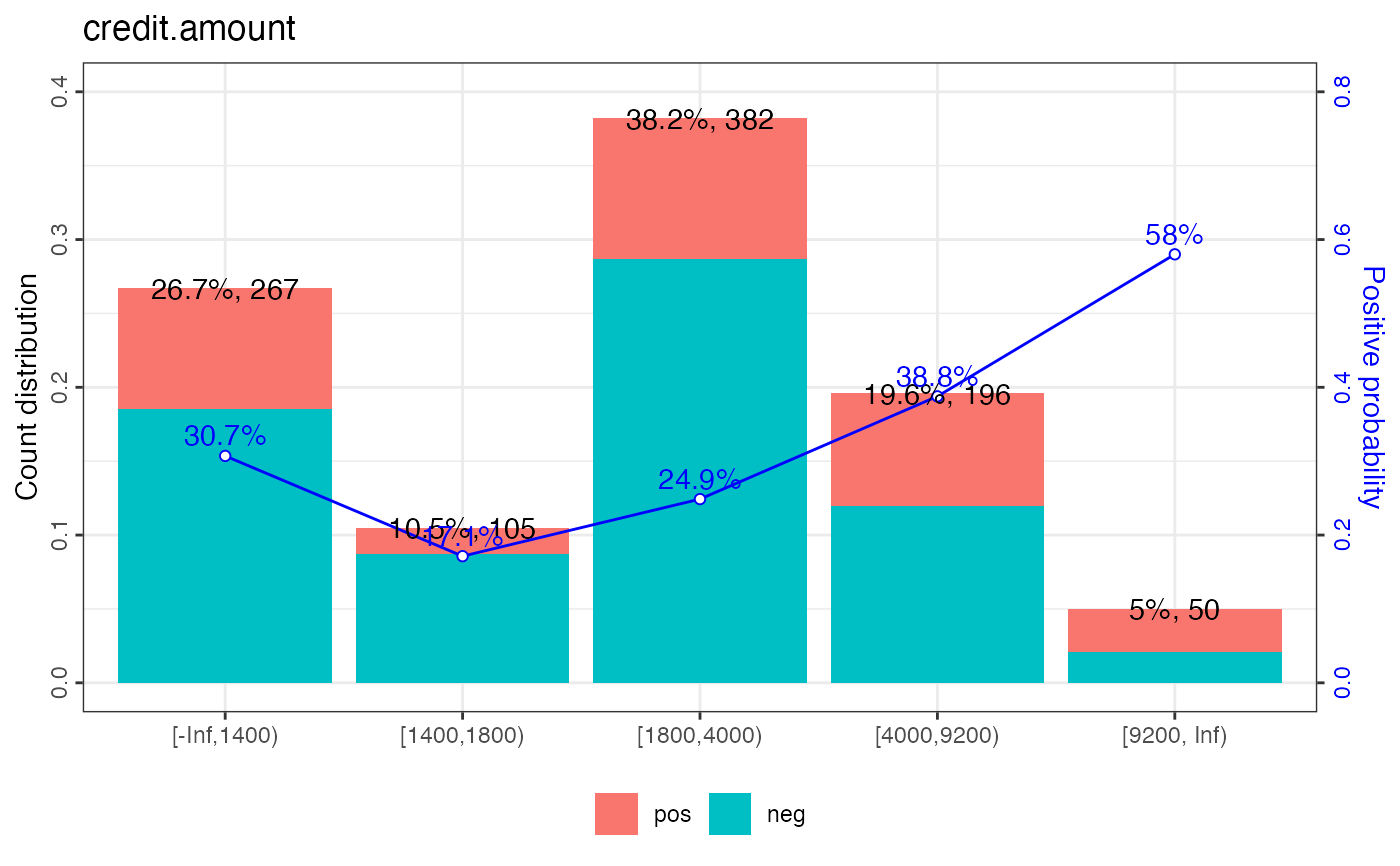#>
p1_lineval = woebin_plot(bins1, show_lineval = FALSE)
print(p1_lineval)
#> $credit.amount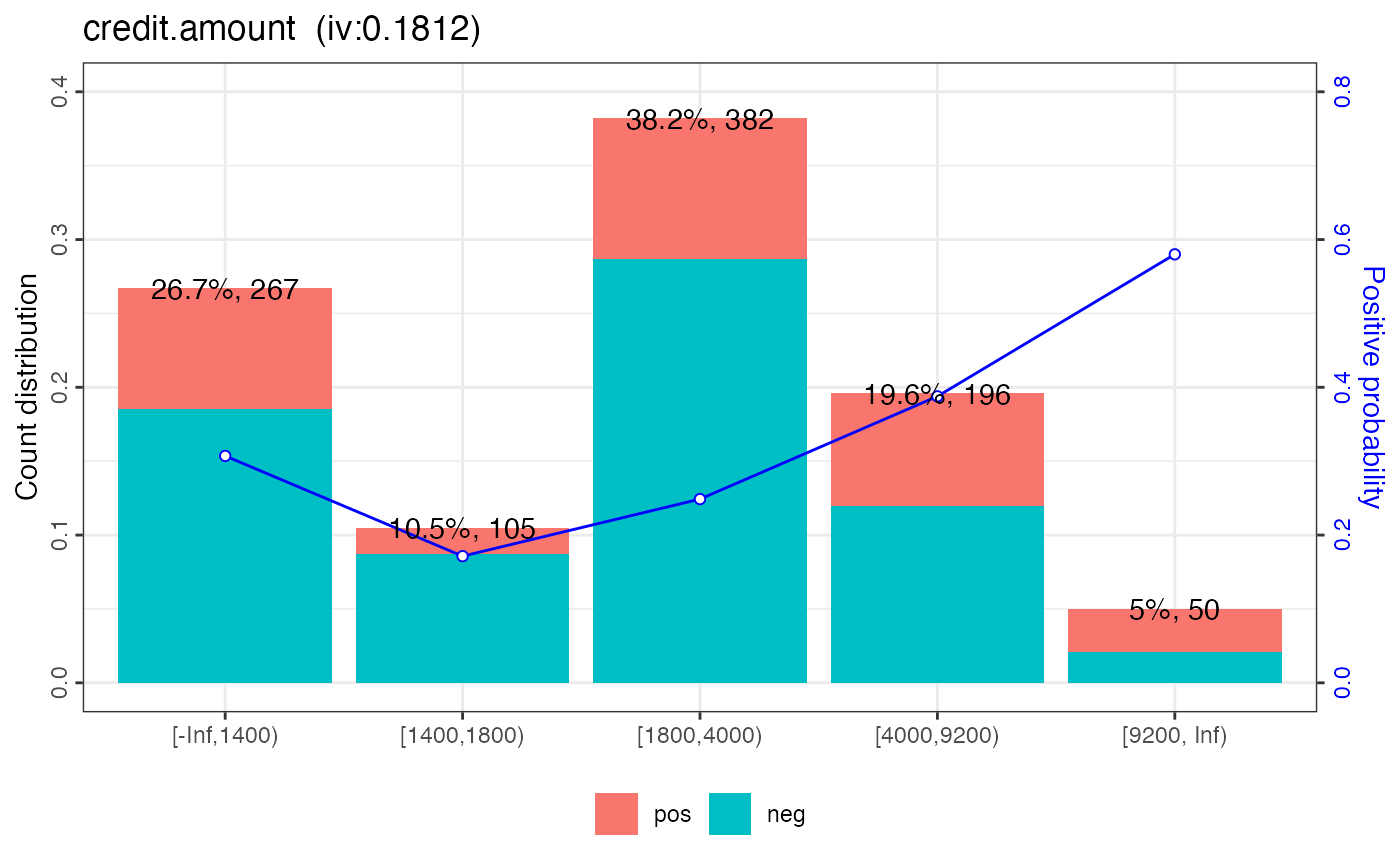#> p1_barval = woebin_plot(bins1, show_barval = FALSE) print(p1_barval) #>$credit.amount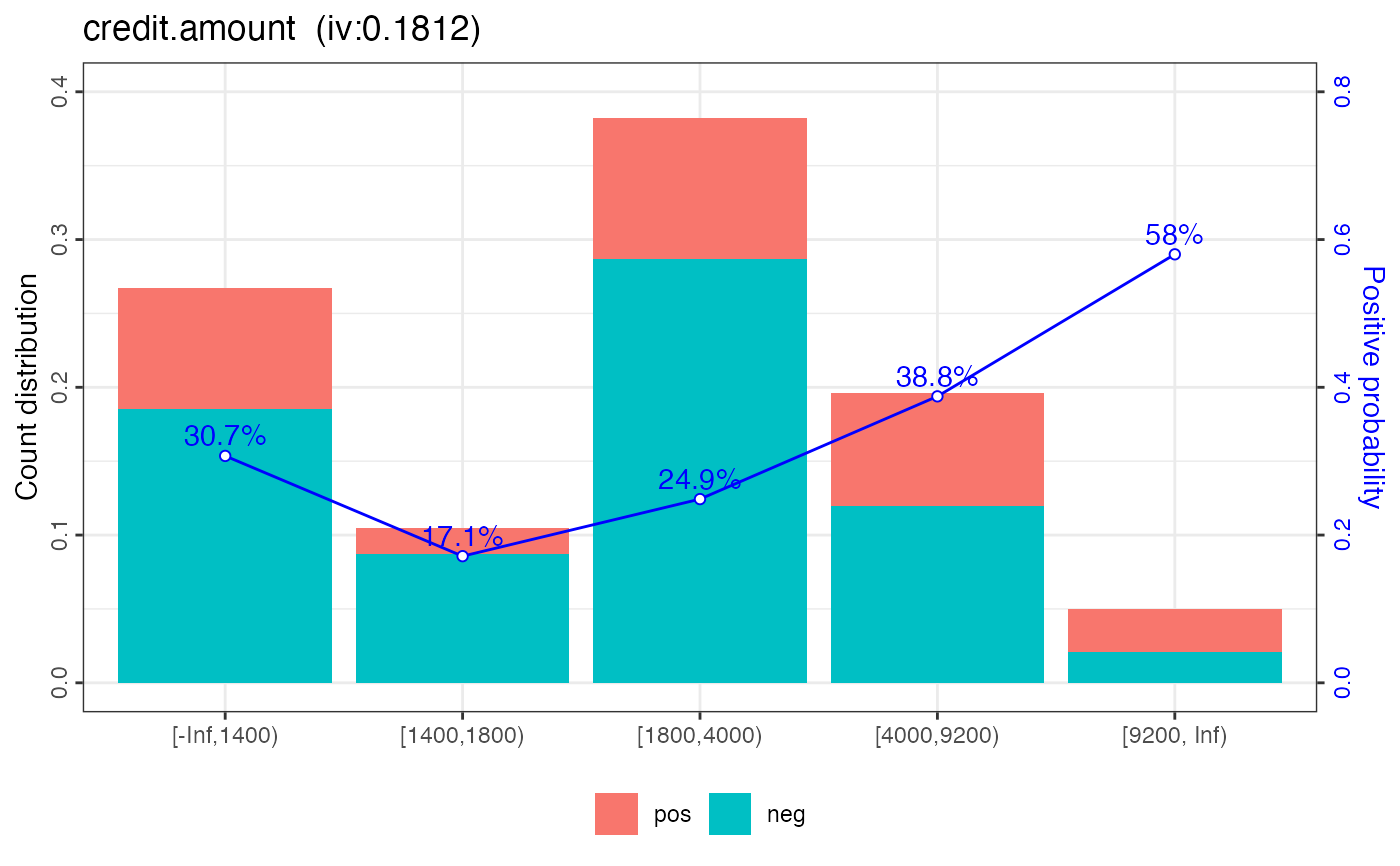#>

# \donttest{
# Example II
bins = woebin(germancredit, y="creditability")
#> ℹ Creating woe binning ...
#> ✔ Binning on 1000 rows and 21 columns in 00:00:01
plotlist = woebin_plot(bins)
print(plotlist\$credit.amount)# # save binning plot
# for (i in 1:length(plotlist)) {
#   ggplot2::ggsave(
#      paste0(names(plotlist[i]), ".png"), plotlist[[i]],
#      width = 15, height = 9, units="cm" )
#   }
# }